# 电梯运行方案的计算机模拟优化设计Computer Simulation and Optimization Design of Elevator Operation Plan

• 全文下载: PDF(1608KB)    PP.209-222   DOI: 10.12677/MOS.2018.74025
• 下载量: 452  浏览量: 1,686

This paper solves the design problem of elevator dispatching scheme in hospital outpatient build-ing by computer simulation. This paper analyzes the following three problems: the use of elevators, the transportation efficiency in rush hours, and the complex elevator dispatching problem. This topic combines MATLAB software, mainly using the computer simulation method. The char-acteristic of this paper is to simulate the running process of the elevator with greater randomness, which greatly reduces the difficulty of solving the problem. This paper not only evaluates the ex-isting elevator operation scheme, but also gives a better design for the elevator operation man-agement scheme in high-rise commercial building, and provides a theoretical basis for it.

1. 引言

2. 电梯调度问题提出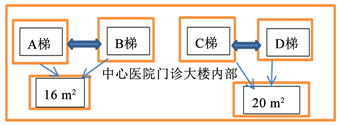Figure 1. Floor plan of the outpatient building of Xuzhou Central Hospital

3. 模型假设

4. 符号说明

${T}_{w}$ ：人均等电梯时间；

${\stackrel{¯}{T}}_{w}$ ：大规模仿真的平均人均等电梯时间；

$N$ ：停靠次数；

$\stackrel{¯}{N}$ ：大规模仿真的平均停靠次数；

${f}_{W}\left({T}_{w}\right)$ ：人均等待电梯时间不满意度隶属值；

${f}_{N}\left(N\right)$ ：平均停靠次数不满意度隶属值；

$\mu$ ：电梯承载率；

${T}_{End}$ ：该电梯当前批次的最终出发时间，也就是当前批次最后一个人的到达时间；

${L}_{End}$ ：该电梯当前批次的最大出发楼层，也就是电梯需要运行的最高位置，到达后即返回。

5. 概念引入及问题分析

5.1. 概念的引入

5.2. 问题分析

6. 模型的建立与求解

6.1. 模型准备与分析

6.1.1. 电梯停靠方案Table 1. Each elevator parking mode

6.1.2. 高峰时刻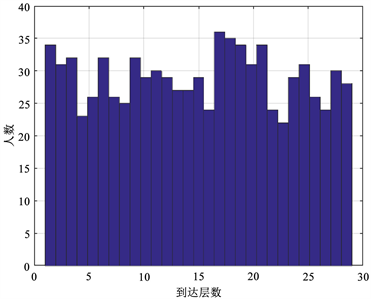(a)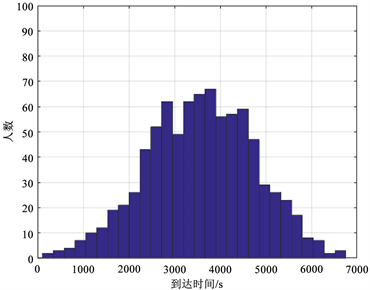(b)

Figure 2. Data distribution: (a) Arrival time distribution map; (b) Map of arrival levels

6.1.3. 电梯运行过程

Step 1：电梯开门，排在前面的一定数量人依次进入电梯，电梯门关闭，向上行驶；

Step 2：判断第i层是否有人下。若无，则不在第i层停靠，电梯驶向第i + 1层。若有，则电梯在第i层停靠，开门，要出人员依次走出电梯，关门。令i = i + 1，判断电梯是否达到最高期望楼层，若否向Step 2开头，若是则转向Step 3；

Step 3：电梯停靠在最高期望楼层，电梯中人员已全部下完，驶向靠在一层。

6.1.4. 方案设计思路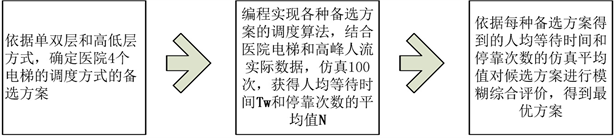Figure 3. Design flow chart of elevator dispatching plan

6.2. 问题1——电梯调度方案评价指标

6.2.1. 评价指标

$评价指标\left\{\begin{array}{cc}\begin{array}{l}满足乘客需求：\\ 节约能源：\end{array}& \begin{array}{l}平均等待时间\\ 停靠次数\end{array}\end{array}$

6.2.2. 电梯调度方案模糊综合评价方法

1) 平均等待指标的隶属化Table 2. Dissatisfaction with waiting time

$f\left(x\right)=\left\{\begin{array}{l}a{\left(x+b\right)}^{2},1\le x\le 3\\ c\mathrm{ln}x+d,3\le x\le 5\end{array}$

a) 当很满意时，隶属度为0，即 ${f}_{W}\left(200\right)=0$

b) 当较满意时，隶属度为0.8，即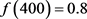c) 当很不满意时，隶属度为1，即 $f\left(1000\right)=1$韩中庚. 数学建模方法及其应用[M]. 第二版. 北京: 高等教育出版社, 2009.  张海龙, 高东红. 几种电梯运行模式的比较及应用[J]. 数学的实践与认识, 2008(10): 65-69.  李庆民, 刘君, 张志华, 武器系统仿真模型的可信性验证方法研究[J]. 系统仿真学报, 2006(12).